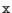Next: .temp Line Up: Initialization Previous: Simulation Options   Contents   Index

## .table Line

General Form:
.table name [ac] x0 v0 x1 v1 ... xN vN
Examples:
.table tab1 0 .1 1n .2 2n .4 3n .2 4n .1 5n 0
.table xgain 0 0 1 1 1 1.5 4 2
.table acvals ac 0 1.0 0, 1e3 .98 .03, ...
.table zz (0 table xgain 4 2)
.table tab1 0 1 .2 .5 .4 table txx .8 .5e-2

The .table line defines a tabulation of data points which can be referenced from other lines in the SPICE file. The data are listed in sequence with respect to the ordinate xN . The elements are separated by white space or commas, and may optionally be surrounded by parentheses. Generally, the table construct consists of many lines, using the `+' or backslash line continuation mechanism. When a table is referenced, the data value returned is interpolated from the values in the table.

The xi in the .table line are values of the independent variable (i.e., the variable given as an argument to the referencing function). The vi entries can be numbers, or a reference to another table in the form

table subtab_name
in which case that table will be referenced to resolve the data point.

If the ac keyword is given, the data numbers vi are expected to be complex values, which are expressed as two values; the real value followed by the imaginary value. Any sub-tables referenced must also have the ac keyword given. The ac tables provide data for frequency-domain analysis. Without ac, all values are real, and the table is intended for use in dc or transient analysis.

A non-ac table is referenced through a tran-function (see 2.15.3). Tables with the ac keyword given are referenced through the ac keyword in dependent and independent sources (see 2.15 and 2.15.4).

Let x be the input variable associated with the device referencing a table. The table is evaluated as follows:

 x < x0 val = v0(x0) x0 << x1 val = v0() if v0 is a table val = interpolation of v0(x0) and v1(x1) if v0 is a number ... x > xN val = vN() if vN is a table val = vN if vN a number val = vN-1(xN) if vN is omitted

See the section A.3 for sample input files which illustrate the use of the .table line.Next: .temp Line Up: Initialization Previous: Simulation Options   Contents   Index
Stephen R. Whiteley 2021-01-27